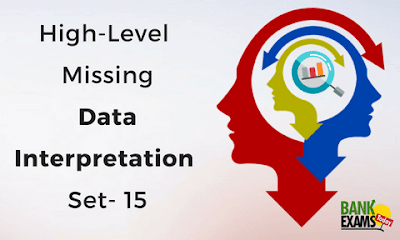New Students Offer - Use Code HELLO

# High Level Missing Data Interpretation Set- 16Direction (Q.1-5): This following table is related to profit and loss and some values are missing. All the discounts are on the M.P. and the Profits are on C.P. Read the Questions carefully and answer the question:

Article
Cost Price (RS)
Profit (%)
Marked Price (Rs)
Discount (%)
Selling Price (Rs)
M
2160
-
2560
-
-
N
-
20%
-
-
-
O
-
-
2875
20%
-
P
1800
-
-
-
1980
Q
-
25%
4000
-
-

### Question. 1

If the ratio of the C.P. of the Article M and Article Q is 3:4, find out the discount % of Article of Q.
A. 12%
B. 15%
C. 8%
D. 10%
E. 20%
Ans. D.
Explanation:
The ratio of the C.P. of the Article M and Article Q is 3:4.
C.P of Article M = 2160 Rs.
C.P. of Article Q = 2160×4/3
=2880 Rs.
S.P. of Article Q = 2880×125%
= 3600 Rs
M.P. of Article Q = 4000 Rs.
Discount % = (M.P.-S.P.)×100/M.P.
= (4000-3600)×100/4000
=10%

### Question. 2

If the discount % and profit % of the Article P is same. Find out the M.P. of Article P.
A. 2800 Rs.
B. 2200 Rs.
C. 3500 Rs.
D. 3000 Rs.
E. None of these.
Ans. B.
Explanation:
The Profit % and the Discount % of the Article P = x
Cost Price×(100+x)/100 = S.P.
1800×(100+x) = 1980×100
180000+1800x = 198000
1800x = 198000-180000
1800x = 18000
x = 10%
M.P. = 1980/90×100
M.P. = 2200 Rs.

### Question. 3

If the M.P. of Article M is Rs. 130 more than the C.P. of Article N and the difference between .P. and S.P. of Article N is Rs. 684. Find the discount % of Article N.
A. 18%
B. 25%
C. 30%
D. 20%
E. None of these.
Ans. E.
Explanation:
M.P. of Article M = 2560 Rs.
C.P. of Article N = 2560-130 = 2430 Rs.
S.P. of Article N = 2430×120/100
= 2916 Rs.
M.P. of Article N = 2916+684 = 3600 Rs.
Discount % = (3600-2916)×100/3600
Discount % = 684×100/3600
Discount % = 19%

### Question. 4

C.P. of Article P is how much % less than the M.P. of Article M?
A. 26%
B. 25.04%
C. 29.68%
D. 31%
E. 28.02%
Ans. C.
Explanation:
C.P of Article P = 1800 Rs.
M.P. of Article M = 2560 Rs.
Less % = (2560-1800)×100/2560
Less % = 760×100/2560
Less % = 29.68%

### Question. 5

The ratio of discount % and profit % is 4:3 of the Article O. Find CP. of Article O.
A. 1200 Rs.
B. 2800 Rs
C. 3500 Rs.
D. 2000 Rs.
E. None of these
Ans. D.
Explanation:
The ratio of discount % and profit % is 4:3.
Discount % = 20%
Profit % = (20×3)/4
= 15%
C.P. = M.P. × (100-Discount %)/ (100+Profit %)
C.P. = 2875 × (100-20%)/ (100+15%)
CP. = 2875×80/115
C.P. = 2000 Rs.